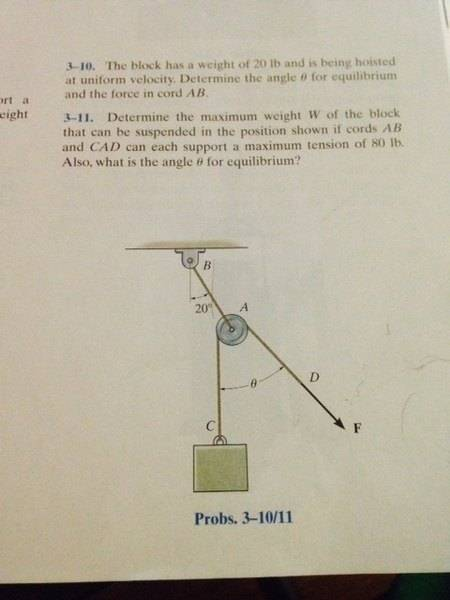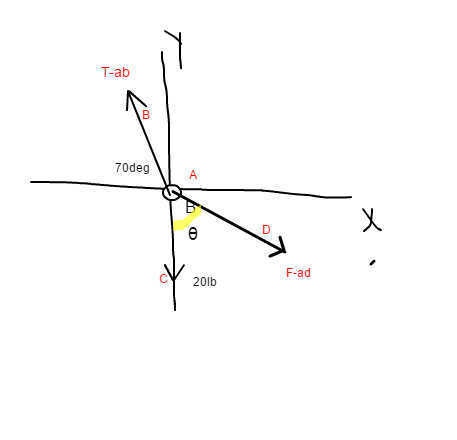# Easy statics problem -- Block being hoisted by a pulley and ropeI drew a FBD about point A and came up with the two equations:
∑Fx=0 20sinθ - T-ab(cos70)=0
ΣFy =0 -20cosθ + T-ab(sin70) -20=0

My answer is not coming out so I either messed up on the FBD or the equations, some help would be appreciated. I'm on 3-10 by the way.

## Answers and Replies

BvU
Science Advisor
Homework Helper
Hard to distinguish what your equations are about; what is the 20 ?
And with T - ab you mean Tab ?
How come W does not feature ? nor the 80 lb ? (lbf ?)

SteamKing
Staff Emeritus
Science Advisor
Homework Helper
Easy statics problem ... which is why it belongs in the Intro Physics HW forum and not one of the technical engineering forums.

Hard to distinguish what your equations are about; what is the 20 ?
And with T - ab you mean Tab ?
How come W does not feature ? nor the 80 lb ? (lbf ?)
Yes that is what I mean. I figured the force at D is 20lb because the block has 20lb of force and the cable holding it has 20lb of tension so D would as well (same cable). So I tried to resolve that 20lbs into the x and y direction.

BvU
Science Advisor
Homework Helper
Sorry, only saw 3-11.
Equations look good. Must be the solution part. What do you do to solve $$\quad 20\sin\theta = T_{AB}\sin(20^\circ) \\ \ 20+ 20 \cos\theta = T_{AB}\cos(20^\circ) \quad {\rm ? }$$

I figured the force at D is 20lb because the block has 20lb of force and the cable holding it has 20lb of tension so D would as well (same cable).
So then your first equation was actually $$F_c = F_f$$ where $$F_c = 20 lbs.$$ So $$F_f = 20 lbs.$$
You probably should have written that equation.

Last edited:
Sorry, only saw 3-11.
Equations look good. Must be the solution part. What do you do to solve $$\quad 20\sin\theta = T_{AB}\sin(20^\circ) \\ \ 20+ 20 \cos\theta = T_{AB}\cos(20^\circ) \quad {\rm ? }$$
I just try solving like any other system of equations. 2 unknowns and 2 equations which is leaving me with funky answers.

What do you get if you solve for the extremes? Sin and cos vary between 0 and 1. You should be able to bracket your answer.

You might as well revise the equations to solve for Tab. Consider replacing the fixed signs with their numbers and divide thru.

Hint: you have a formula error... Sin is hypotenuse over the what? Cos is hypotenuse over the what?

hint2: one of the angles is taken from the horizontal x axis. The other is from the vertical y axis. How does this affect if you use sin or cos...

Last edited:
I figured it out guys, thanks for the help.

I figured it out guys, thanks for the help.
And the answer is what? I got 31.32 with the fixed equations.

Last edited:
And the answer is what? I got 31.32 with the fixed equations.

The angle is 40degrees and Tab=37.6lb

Really, I am getting degrees =34.91 and Tab 33.46? Original equation above did not divide by cos(20)

Hmmm, not very good at using Numbers on an iPad yet. Perhaps I should try it by hand.

Okay. got it. Tab = 37.6 and angle = 40.

Last edited:
I figured it out guys, thanks for the help.

Edit: Add in tension equation

Alternative solution. Rotate your x/y axis so that x is parallel to $$T_{ab}$$

A-C is 20 degree angle from axis.
A-D is unknown angle

$$F_ {ac} = F_{ad}$$

$$F_{ac} cos \theta = F_{ad} cos (uk)$$
And therefore uk angle = theta.

And

$$F_{ab} = F_{ac} sin \theta + F_{ad} sin (uk)$$

which reduces to

$$F_{ab} = 2 * F_{ac} sin \theta$$

$$F_{ab} = 2 * 20 sin (20 degrees)$$

Voila

Last edited:
•Oxford365